# 搞懂视觉 Transformer 原理和代码，看这篇技术综述就够了

Transformer 是 Google 的团队在 2017 年提出的一种 NLP 经典模型，现在比较火热的 Bert 也是基于 Transformer。Transformer 模型使用了 Self-Attention 机制，不采用RNN顺序结构，使得模型可以并行化训练，而且能够拥有全局信息。本文将对Vision Transformer的原理和代码进行非常全面的解读。考虑到每篇文章字数的限制，每一篇文章将按照目录的编排包含三个小节，而且这个系列会随着Vision Transformer的发展而长期更新。

## 目录

(每篇文章对应一个Section，目录持续更新。)

• Section 1

1 一切从Self-attention开始
1.1 处理Sequence数据的模型
1.2 Self-attention
1.4 Positional Encoding

2 Transformer的实现和代码解读 (NIPS2017)
2.1 Transformer原理分析
2.2 Transformer代码解读

3 Transformer+Detection：引入视觉领域的首创DETR (ECCV2020)
3.1 DETR原理分析
3.2 DETR代码解读

• Section 2

4 Transformer+Detection：Deformable DETR：可变形的Transformer (ICLR2021)
(来自商汤代季峰老师组)
4.1 Deformable DETR原理分析
4.2 Deformable DETR代码解读

5 Transformer+Classification：用于分类任务的Transformer
(ICLR2021)

5.1 ViT原理分析
5.2 ViT代码解读

6 Transformer+Image Processing：IPT：用于底层视觉任务的Transformer
(来自北京华为诺亚方舟实验室)
6.1 IPT原理分析

• Section 3

7 Transformer+Segmentation：SETR：基于Transformer 的语义分割
(来自复旦大学，腾讯优图等)
7.1 SETR原理分析

8 Transformer+GAN：VQGAN：实现高分辨率的图像生成
(来自德国海德堡大学)
8.1 VQGAN原理分析
8.2 VQGAN代码解读

9 Transformer+Distillation：DeiT：高效图像Transformer
9.1 DeiT原理分析

Transformer 是 Google 的团队在 2017 年提出的一种 NLP 经典模型，现在比较火热的 Bert 也是基于 Transformer。Transformer 模型使用了 Self-Attention 机制，不采用 RNN 的

，使得模型可以并行化训练，而且能够拥有全局信息。

## 1 一切从Self-attention开始

• 1.1 处理Sequence数据的模型：

Transformer是一个Sequence to Sequence model，特别之处在于它大量用到了self-attention。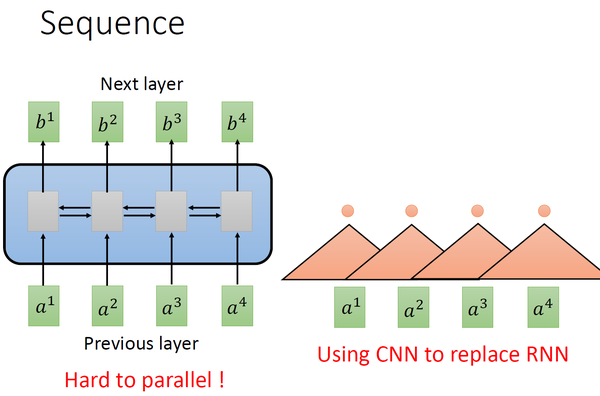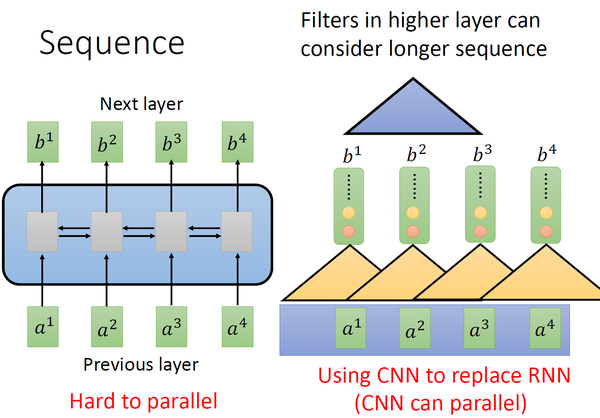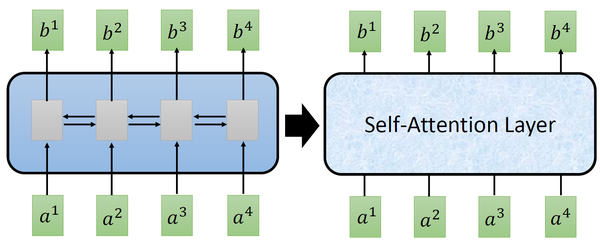• 1.2 Self-attention：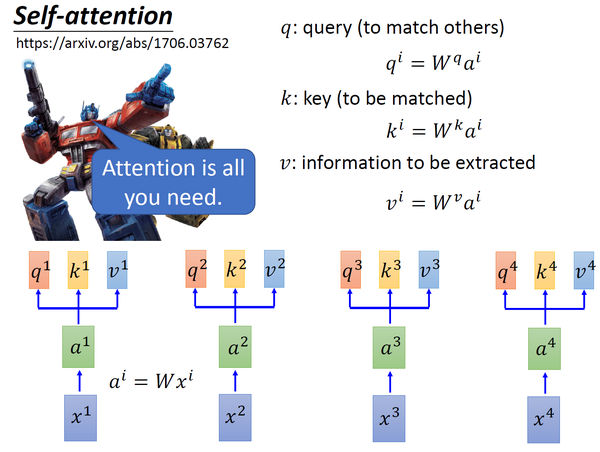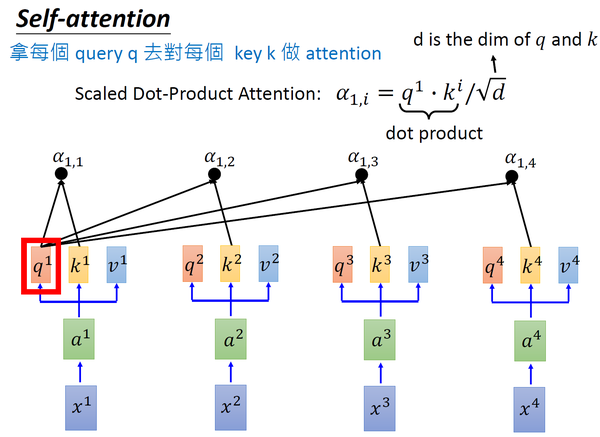$$\alpha_{1,i}=q^1\cdot k^i/\sqrt{d} \tag{1}$$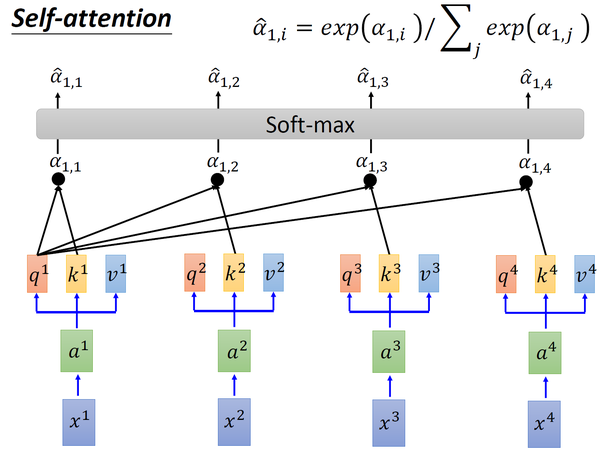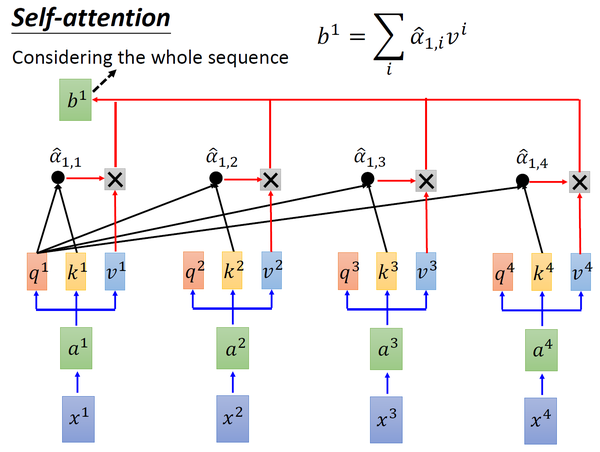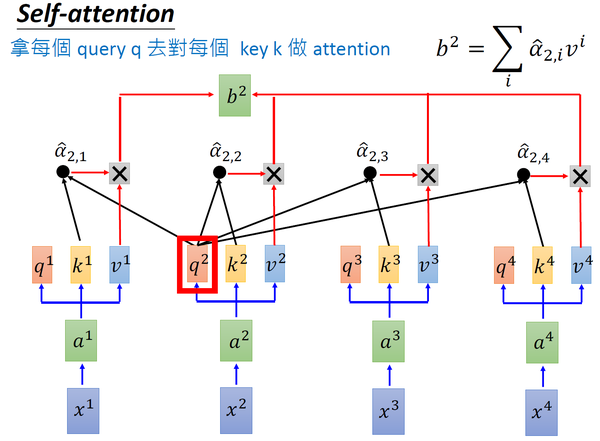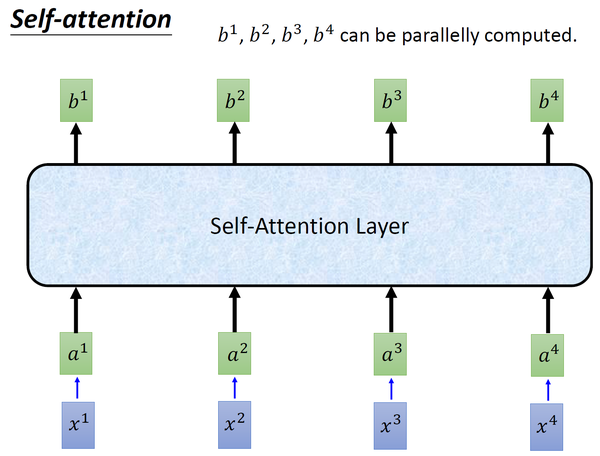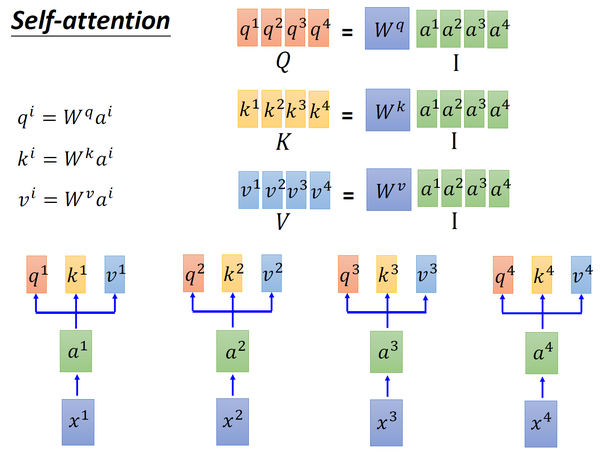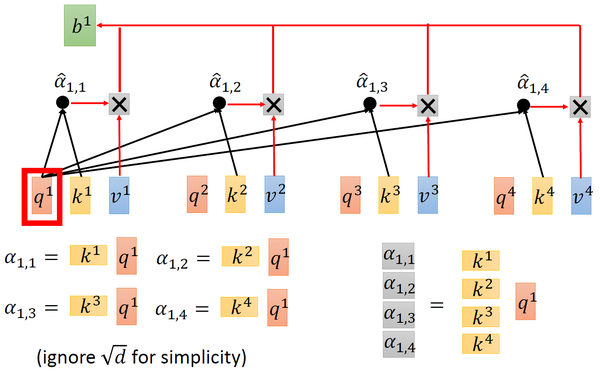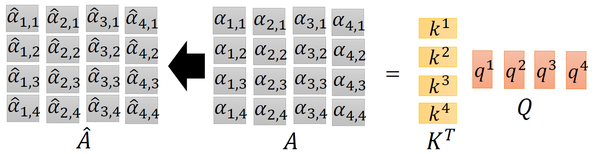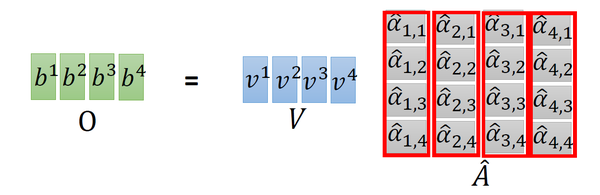$$\hat A=\text{softmax}(A)=K^T\cdot Q \tag{2}$$

$$O=V\cdot\hat A\tag{3}$$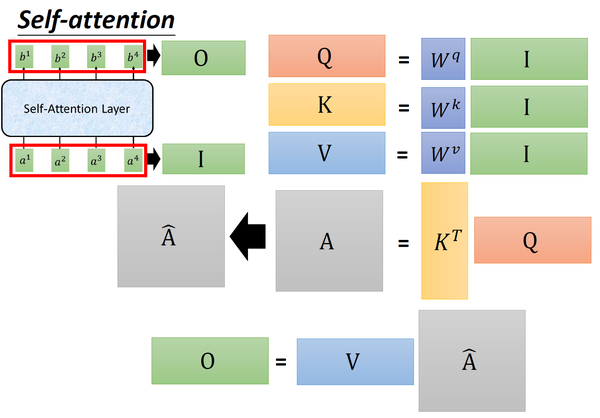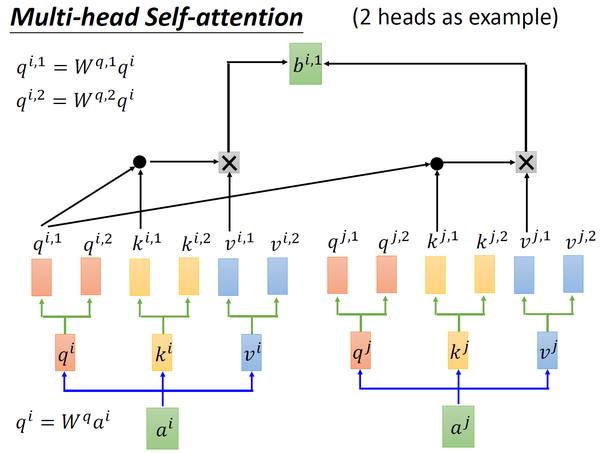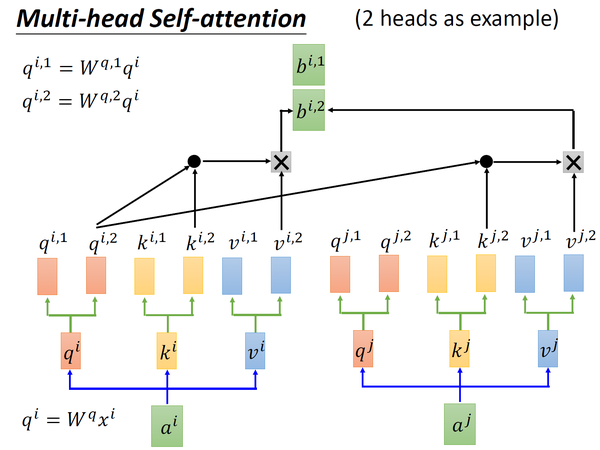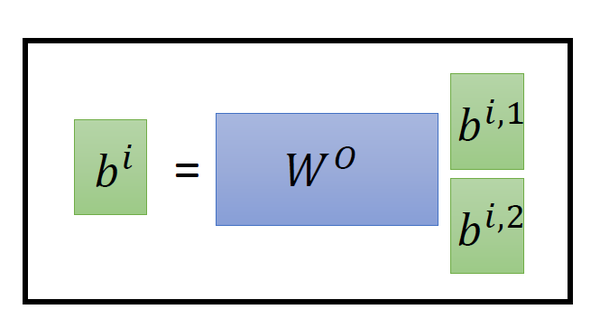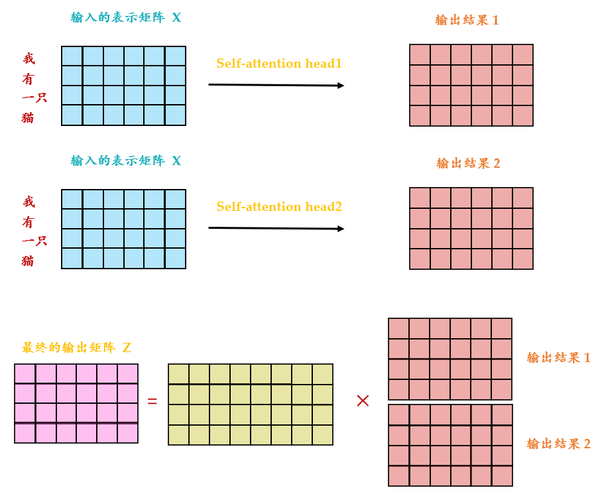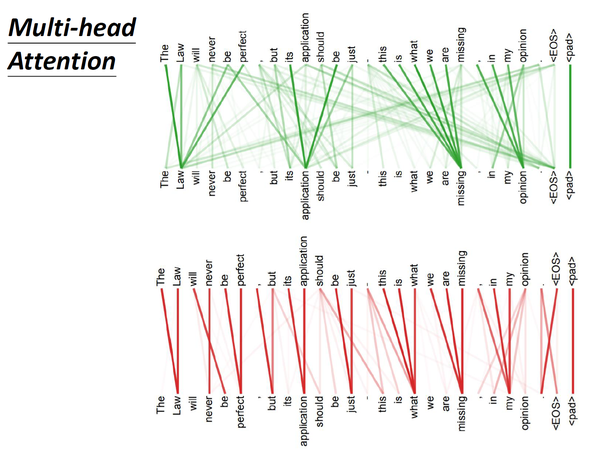• 1.4 Positional Encoding：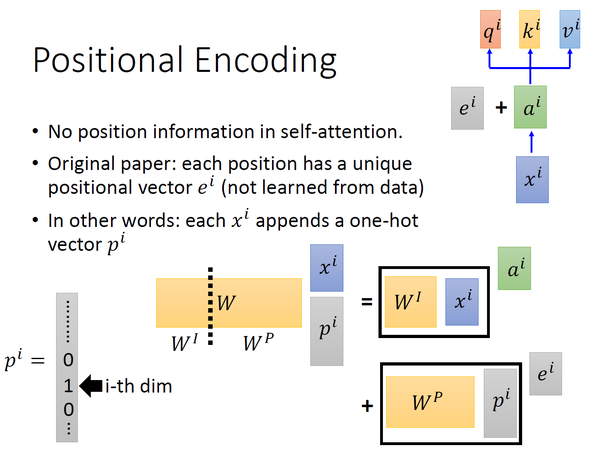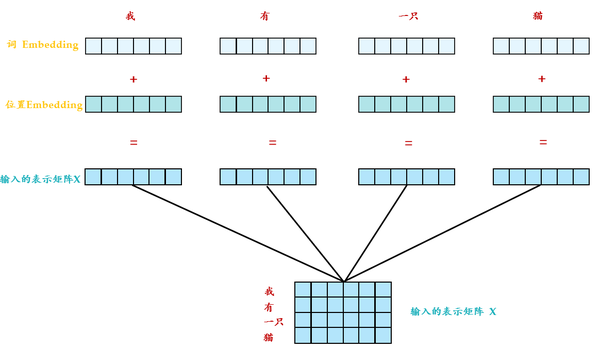$$W\cdot x_p^i=[W^I,W^P]\cdot\begin{bmatrix}x^i\p^i \end{bmatrix}=W^I\cdot x^i+W^P\cdot p^i=a^i+e^i \tag{4}$$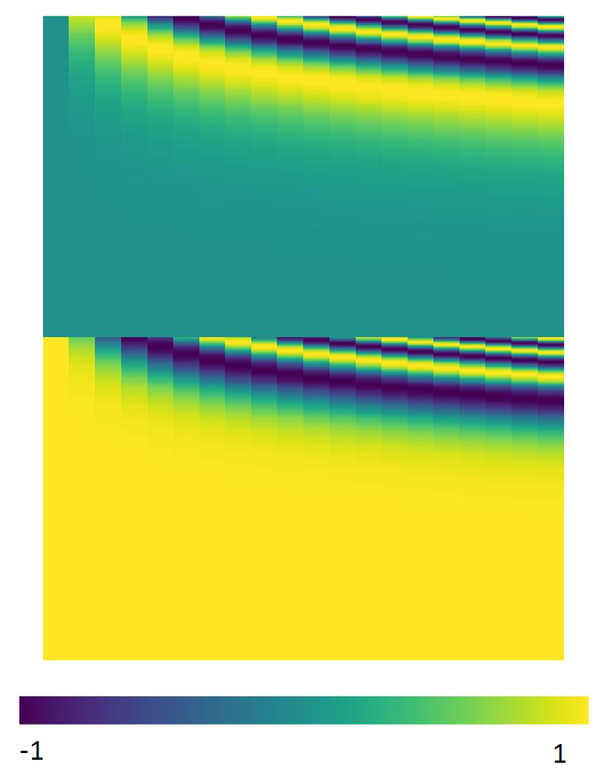Transformer 中除了单词的 Embedding，还需要使用位置 Embedding 表示单词出现在句子中的位置。因为 Transformer 不采用 RNN 的结构，而是使用全局信息，不能利用单词的顺序信息，而这部分信息对于 NLP 来说非常重要。所以 Transformer 中使用位置 Embedding 保存单词在序列中的相对或绝对位置。

\begin{align}PE{(pos, 2i)} = sin(pos/10000^{2i/d{model}}) \ PE{(pos, 2i+1)} = cos(pos/10000^{2i/d{model}}) \end{align}\tag{5}

$i$ ，或者准确意义上是 $2i$ 和 $2i+1$ 表示了Positional Encoding的维度，$i$ 的取值范围是： $\left[ 0,\ldots ,{{{d}_{model}}}/{2}\; \right)$ 。所以当 $pos$ 为1时，对应的Positional Encoding可以写成：

$$PE\left( 1 \right)=\left[ \sin \left( {1}/{{{10000}^{{0}/{512}\;}}}\; \right),\cos \left( {1}/{{{10000}^{{0}/{512}\;}}}\; \right),\sin \left( {1}/{{{10000}^{{2}/{512}\;}}}\; \right),\cos \left( {1}/{{{10000}^{{2}/{512}\;}}}\; \right),\ldots \right]$$

• 每个位置有一个唯一的positional encoding。
• 使 $PE$ 能够适应比训练集里面所有句子更长的句子，假设训练集里面最长的句子是有 20 个单词，突然来了一个长度为 21 的句子，则使用公式计算的方法可以计算出第 21 位的 Embedding。
• 可以让模型容易地计算出相对位置，对于固定长度的间距 $k$ ，任意位置的 $PE{pos+k}$ 都可以被 $PE{pos}$ 的线性函数表示，因为三角函数特性：

$$cos(\alpha+\beta) = cos(\alpha)cos(\beta)-sin(\alpha)sin(\beta) \$$

$$sin(\alpha+\beta) = sin(\alpha)cos(\beta) + cos(\alpha)sins(\beta) \$$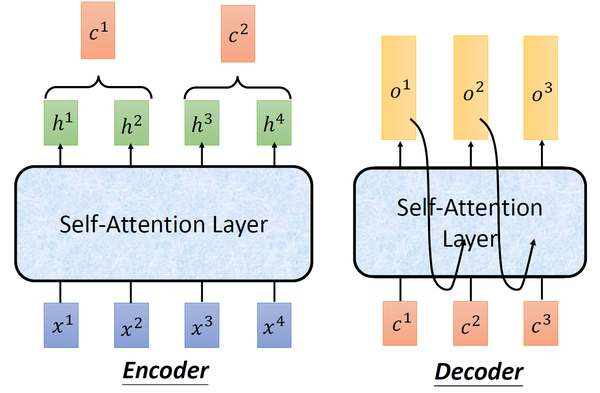## 2 Transformer的实现和代码解读

• 2.1 Transformer原理分析：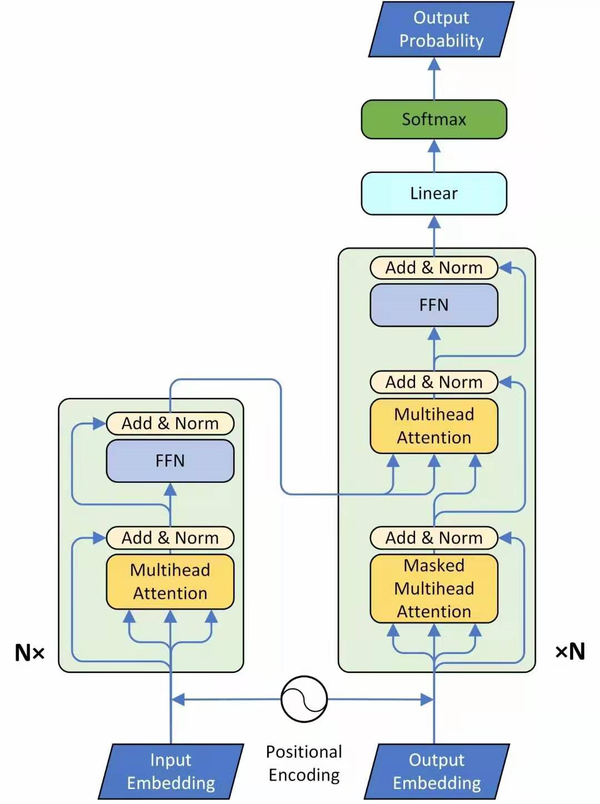Encoder：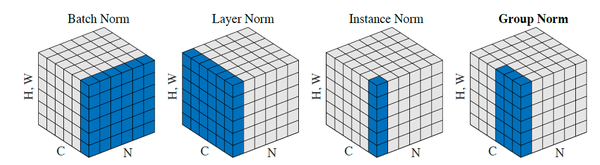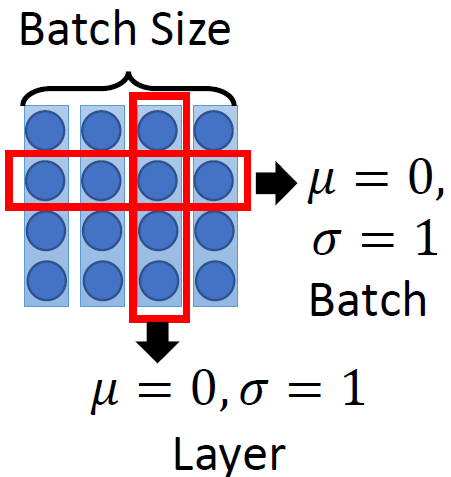$$\color{darkgreen}{O_1}=\color{green}{\text{Layer Normalization}}(\color{teal}{I}+\color{crimson}{\text{Multi-head Self-Attention}}(\color{teal}{I}))\tag{6}$$

$$\color{darkgreen}{O_2}=\color{green}{\text{Layer Normalization}}(\color{teal}{O_1}+\color{crimson}{\text{Feed Forward Network}}(\color{teal}{O_1}))\tag{7}$$

$$\color{green}{\text{Block}}(\color{teal}{I})=\color{green}{O_2} \tag{8}$$

$$\color{purple}{\text{Encoder}}(\color{darkgreen}{I})=\color{darkgreen}{\text{Block}}(...\color{darkgreen}{\text{Block}}(\color{darkgreen}{\text{Block}})(\color{teal}{I}))\\quad N\;times \tag{9}$$

Decoder：

$\color{crimson}{i-1}$

。所以中间的attention不是self-attention，它的Key和Value来自encoder，Query来自上一位置 $\color{crimson}{Decoder}$ 的输出。

，因为要用上一个位置的输入当作attention的query。

• 第二个 Multi-Head Attention 层的Key，Value矩阵使用 Encoder 的编码信息矩阵 $C$ 进行计算，而Query使用上一个 Decoder block 的输出计算。
• 最后有一个 Softmax 层计算下一个翻译单词的概率。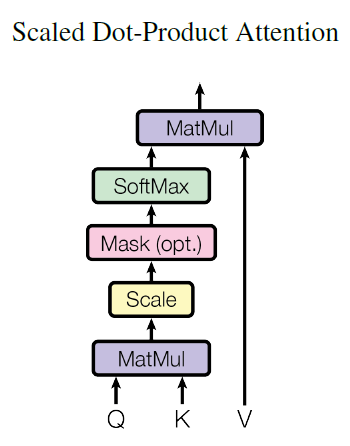Decoder 可以在训练的过程中使用 Teacher Forcing 并且并行化训练，即将正确的单词序列 (\ I have a cat) 和对应输出 (I have a cat \) 传递到 Decoder。那么在预测第 $i$

$i+1$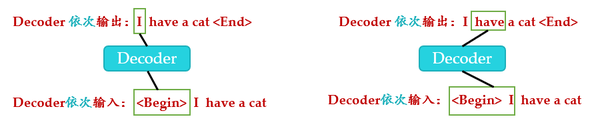1. 输入\，解码器输出 I 。
2. 输入前面已经解码的\和 I，解码器输出have。
3. 输入已经解码的\，I, have, a, cat，解码器输出解码结束标志位\，每次解码都会利用前面已经解码输出的所有单词嵌入信息。

Transformer测试时的解码过程：

。要实现这个功能就可以参考编码器的操作，把目标单词嵌入向量组成矩阵一次输入即可。即：并行化训练。

Step1： 输入矩阵包含 "\ I have a cat" (0, 1, 2, 3, 4) 五个单词的表示向量，Mask是一个 5×5 的矩阵。在Mask可以发现单词 0 只能使用单词 0 的信息，而单词 1 可以使用单词 0, 1 的信息，即只能使用之前的信息。输入矩阵 $X\in R_{N,dx}$ 经过transformation matrix变为3个矩阵：Query $Q\in R{N,d}$ ，Key $K\in R{N,d}$ 和Value $V\in R{N,d}$ 。

Step2： $Q^T\cdot K$ 得到 Attention矩阵 $A\in R{N,N}$ ，此时先不急于做softmax的操作，而是先于一个 $\text{Mask}\in R{N,N}$ 矩阵相乘，使得attention矩阵的有些位置 归0，得到Masked Attention矩阵 $\text{Mask Attention}\in R{N,N}$ 。 $\text{Mask}\in R{N,N}$ 矩阵是个下三角矩阵，为什么这样设计？是因为想在计算 $Z$ 矩阵的某一行时，只考虑它前面token的作用。即：在计算 $Z$ 的第一行时，刻意地把 $\text{Attention}$ 矩阵第一行的后面几个元素屏蔽掉，只考虑 $\text{Attention}_{0,0}$ 。在产生have这个单词时，只考虑 I，不考虑之后的have a cat，即只会attend on已经产生的sequence，这个很合理，因为还没有产生出来的东西不存在，就无法做attention。

Step3： Masked Attention矩阵进行 Softmax，每一行的和都为 1。但是单词 0 在单词 1, 2, 3, 4 上的 attention score 都为 0。得到的结果再与 $V$ 矩阵相乘得到最终的self-attention层的输出结果 $Z1\in R{N,d}$ 。

Step4： $Z1\in R{N,d}$ 只是某一个head的结果，将多个head的结果concat在一起之后再最后进行Linear Transformation得到最终的Masked Multi-Head Self-attention的输出结果 $Z\in R_{N,d}$ 。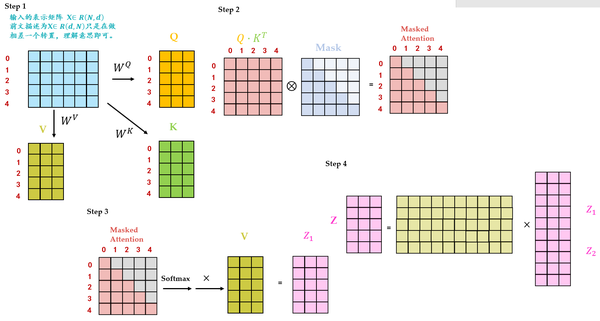$\text{Key, Value}$ 来自Transformer Encoder的输出，所以可以看做句子(Sequence)/图片(image)内容信息(content，比如句意是："我有一只猫"，图片内容是："有几辆车，几个人等等")

$\text{Query}$ 表达了一种诉求：希望得到什么，可以看做引导信息(guide)

Decoder的最后是Softmax 预测输出单词。因为 Mask 的存在，使得单词 0 的输出 $Z(0,)$ 只包含单词 0 的信息。Softmax 根据输出矩阵的每一行预测下一个单词，如下图25所示。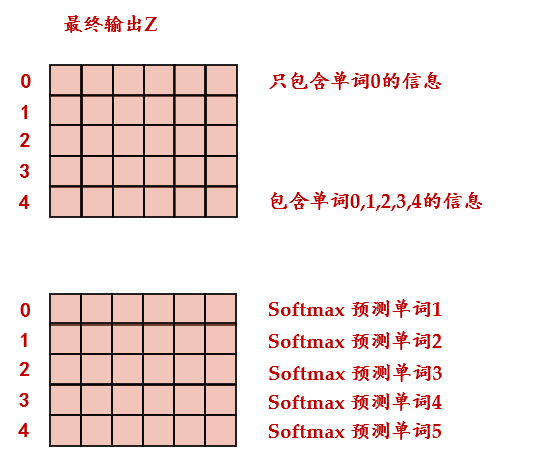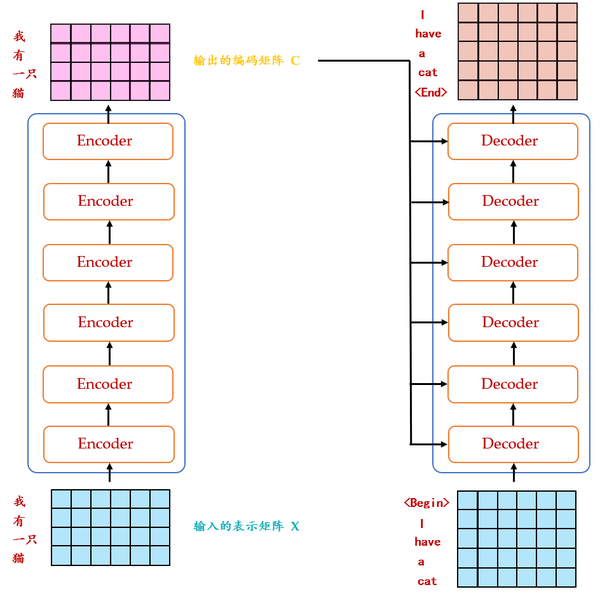• 2.2 Transformer代码解读：

ScaledDotProductAttention：

class ScaledDotProductAttention(nn.Module):
''' Scaled Dot-Product Attention '''

def __init__(self, temperature, attn_dropout=0.1):
super().__init__()
self.temperature = temperature
self.dropout = nn.Dropout(attn_dropout)

def forward(self, q, k, v, mask=None):

attn = torch.matmul(q / self.temperature, k.transpose(2, 3))

attn = self.dropout(F.softmax(attn, dim=-1))
output = torch.matmul(attn, v)

return output, attn

class PositionalEncoding(nn.Module):

def __init__(self, d_hid, n_position=200):
super(PositionalEncoding, self).__init__()

# Not a parameter
self.register_buffer('pos_table', self._get_sinusoid_encoding_table(n_position, d_hid))

def _get_sinusoid_encoding_table(self, n_position, d_hid):
''' Sinusoid position encoding table '''
# TODO: make it with torch instead of numpy

def get_position_angle_vec(position):
return [position / np.power(10000, 2 * (hid_j // 2) / d_hid) for hid_j in range(d_hid)]

sinusoid_table = np.array([get_position_angle_vec(pos_i) for pos_i in range(n_position)])
sinusoid_table[:, 0::2] = np.sin(sinusoid_table[:, 0::2])  # dim 2i
sinusoid_table[:, 1::2] = np.cos(sinusoid_table[:, 1::2])  # dim 2i+1

def forward(self, x):
# x(B,N,d)
return x + self.pos_table[:, :x.size(1)].clone().detach()

class MultiHeadAttention(nn.Module):

def __init__(self, n_head, d_model, d_k, d_v, dropout=0.1):
super().__init__()

self.d_k = d_k
self.d_v = d_v

self.w_qs = nn.Linear(d_model, n_head * d_k, bias=False)
self.w_ks = nn.Linear(d_model, n_head * d_k, bias=False)
self.w_vs = nn.Linear(d_model, n_head * d_v, bias=False)
self.fc = nn.Linear(n_head * d_v, d_model, bias=False)

self.attention = ScaledDotProductAttention(temperature=d_k **
0.5)

self.dropout = nn.Dropout(dropout)
self.layer_norm = nn.LayerNorm(d_model, eps=1e-6)

def forward(self, q, k, v, mask=None):

sz_b, len_q, len_k, len_v = q.size(0), q.size(1), k.size(1), v.size(1)

residual = q

# Pass through the pre-attention projection: b x lq x (n*dv)
# Separate different heads: b x lq x n x dv
q = self.w_qs(q).view(sz_b, len_q, n_head, d_k)
k = self.w_ks(k).view(sz_b, len_k, n_head, d_k)
v = self.w_vs(v).view(sz_b, len_v, n_head, d_v)

# Transpose for attention dot product: b x n x lq x dv
q, k, v = q.transpose(1, 2), k.transpose(1, 2), v.transpose(1, 2)

# Transpose to move the head dimension back: b x lq x n x dv
# Combine the last two dimensions to concatenate all the heads together: b x lq x (n*dv)
q = q.transpose(1, 2).contiguous().view(sz_b, len_q, -1)

q = self.dropout(self.fc(q))
q += residual

q = self.layer_norm(q)

return q, attn

class PositionwiseFeedForward(nn.Module):
''' A two-feed-forward-layer module '''

def __init__(self, d_in, d_hid, dropout=0.1):
super().__init__()
self.w_1 = nn.Linear(d_in, d_hid) # position-wise
self.w_2 = nn.Linear(d_hid, d_in) # position-wise
self.layer_norm = nn.LayerNorm(d_in, eps=1e-6)
self.dropout = nn.Dropout(dropout)

def forward(self, x):

residual = x

x = self.w_2(F.relu(self.w_1(x)))
x = self.dropout(x)
x += residual

x = self.layer_norm(x)

return x

EncoderLayer：

class EncoderLayer(nn.Module):
''' Compose with two layers '''

def __init__(self, d_model, d_inner, n_head, d_k, d_v, dropout=0.1):
super(EncoderLayer, self).__init__()
self.pos_ffn = PositionwiseFeedForward(d_model, d_inner, dropout=dropout)

enc_output, enc_slf_attn = self.slf_attn(
enc_output = self.pos_ffn(enc_output)
return enc_output, enc_slf_attn

DecoderLayer：

class DecoderLayer(nn.Module):
''' Compose with three layers '''

def __init__(self, d_model, d_inner, n_head, d_k, d_v, dropout=0.1):
super(DecoderLayer, self).__init__()
self.pos_ffn = PositionwiseFeedForward(d_model, d_inner, dropout=dropout)

def forward(
self, dec_input, enc_output,
dec_output, dec_slf_attn = self.slf_attn(
dec_output, dec_enc_attn = self.enc_attn(
dec_output = self.pos_ffn(dec_output)
return dec_output, dec_slf_attn, dec_enc_attn

Encoder：

class Encoder(nn.Module):
''' A encoder model with self attention mechanism. '''

def __init__(
self, n_src_vocab, d_word_vec, n_layers, n_head, d_k, d_v,

super().__init__()

self.position_enc = PositionalEncoding(d_word_vec, n_position=n_position)
self.dropout = nn.Dropout(p=dropout)
self.layer_stack = nn.ModuleList([
EncoderLayer(d_model, d_inner, n_head, d_k, d_v, dropout=dropout)
for _ in range(n_layers)])
self.layer_norm = nn.LayerNorm(d_model, eps=1e-6)

enc_slf_attn_list = []

# -- Forward

enc_output = self.dropout(self.position_enc(self.src_word_emb(src_seq)))
enc_output = self.layer_norm(enc_output)

for enc_layer in self.layer_stack:
enc_slf_attn_list += [enc_slf_attn] if return_attns else []

if return_attns:
return enc_output, enc_slf_attn_list
return enc_output,

Decoder：

class Decoder(nn.Module):
''' A decoder model with self attention mechanism. '''

dec_slf_attn_list, dec_enc_attn_list = [], []

# -- Forward
dec_output = self.dropout(self.position_enc(self.trg_word_emb(trg_seq)))
dec_output = self.layer_norm(dec_output)

for dec_layer in self.layer_stack:
dec_output, dec_slf_attn, dec_enc_attn = dec_layer(
dec_slf_attn_list += [dec_slf_attn] if return_attns else []
dec_enc_attn_list += [dec_enc_attn] if return_attns else []

if return_attns:
return dec_output, dec_slf_attn_list, dec_enc_attn_list
return dec_output,

class Transformer(nn.Module):
''' A sequence to sequence model with attention mechanism. '''

def __init__(
d_word_vec=512, d_model=512, d_inner=2048,
n_layers=6, n_head=8, d_k=64, d_v=64, dropout=0.1, n_position=200,
trg_emb_prj_weight_sharing=True, emb_src_trg_weight_sharing=True):

super().__init__()

self.encoder = Encoder(
n_src_vocab=n_src_vocab, n_position=n_position,
d_word_vec=d_word_vec, d_model=d_model, d_inner=d_inner,

self.decoder = Decoder(
n_trg_vocab=n_trg_vocab, n_position=n_position,
d_word_vec=d_word_vec, d_model=d_model, d_inner=d_inner,

self.trg_word_prj = nn.Linear(d_model, n_trg_vocab, bias=False)

for p in self.parameters():
if p.dim() > 1:
nn.init.xavier_uniform_(p)

assert d_model == d_word_vec, \
'To facilitate the residual connections, \
the dimensions of all module outputs shall be the same.'

self.x_logit_scale = 1.
if trg_emb_prj_weight_sharing:
# Share the weight between target word embedding & last dense layer
self.trg_word_prj.weight = self.decoder.trg_word_emb.weight
self.x_logit_scale = (d_model **
-0.5)

if emb_src_trg_weight_sharing:
self.encoder.src_word_emb.weight = self.decoder.trg_word_emb.weight

def forward(self, src_seq, trg_seq):

seq_logit = self.trg_word_prj(dec_output) * self.x_logit_scale

return seq_logit.view(-1, seq_logit.size(2))

def get_pad_mask(seq, pad_idx):

''' For masking out the subsequent info. '''
sz_b, len_s = seq.size()
torch.ones((1, len_s, len_s), device=seq.device), diagonal=1)).bool()
return subsequent_mask

## 3 Transformer+Detection：引入视觉领域的首创DETR

https://arxiv.org/abs/2005.12872​arxiv.org

• 3.1 DETR原理分析：

$$\color{indianred}{\text{网络架构部分解读:}}$$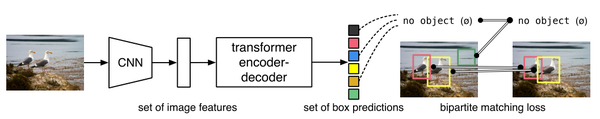$N$

DETR整体结构可以分为四个部分：backbone，encoder，decoder和FFN，如下图28所示，以下分别解释这四个部分：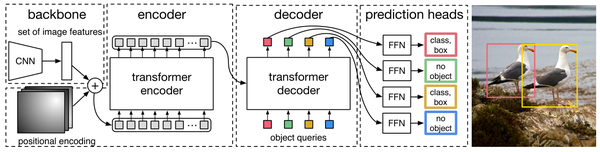1 首先看backbone： CNN backbone处理 $x_{\text{img}}\in B\times 3\times H_0 \times W_0$维的图像，把它转换为$f\in R^{B\times C\times H\times W}$维的feature map（一般来说 $C = 2048或256, H = \frac{H_0}{32}, W = \frac{W_0}{32}$），backbone只做这一件事。

2 再看encoder： encoder的输入是$f\in R^{B\times C\times H\times W}$维的feature map，接下来依次进行以下过程：

• 通道数压缩： 先用 $1\times 1$ convolution处理，将channels数量从 $C$ 压缩到 $d$，即得到$z_0\in R^{B\times d\times H\times W}$维的新feature map。
• 转化为序列化数据： 将空间的维度（高和宽）压缩为一个维度，即把上一步得到的$z_0\in R^{B\times d\times H\times W}(d=256)$维的feature map通过reshape成$(HW,B,256)$维的feature map。
• 位置编码： 在得到了$z_0\in R^{B\times d\times H\times W}$维的feature map之后，正式输入encoder之前，需要进行
Positional Encoding
。这一步在第2节讲解transformer的时候已经提到过，因为
在self-attention中需要有表示位置的信息
，否则你的sequence = "A打了B" 还是sequence = "B打了A"的效果是一样的。但是transformer encoder这个结构本身却无法体现出位置信息。也就是说，我们需要对这个 $z_0\in R^{B\times d\times H\times W}$ 维的feature map做positional encoding。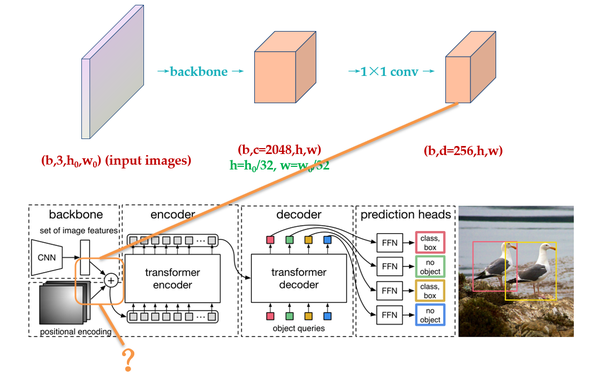\begin{align}PE{(pos, 2i)} = sin(pos/10000^{2i/d}) \ PE{(pos, 2i+1)} = cos(pos/10000^{2i/d}) \end{align}\tag{10}

$i$ ，或者准确意义上是 $2i$ 和 $2i+1$ 表示了Positional Encoding的维度，$i$ 的取值范围是： $\left[ 0,\ldots ,{{{d}}}/{2}\; \right)$ 。所以当 $pos$ 为1时，对应的Positional Encoding可以写成：

$$PE\left( 1 \right)=\left[ \sin \left( {1}/{{{10000}^{{0}/{256}\;}}}\; \right),\cos \left( {1}/{{{10000}^{{0}/{256}\;}}}\; \right),\sin \left( {1}/{{{10000}^{{2}/{256}\;}}}\; \right),\cos \left( {1}/{{{10000}^{{2}/{256}\;}}}\; \right),\ldots \right]$$

Positional Encoding的输出张量是： $(B,d,H,W),d=256$ ，其中 $d$ 代表位置编码的长度， $H,W$ 代表张量的位置。意思是说，这个特征图上的任意一个点 $(H_1,W_1)$ 有个位置编码，这个编码的长度是256，其中，前128维代表 $H_1$ 的位置编码，后128维代表 $W_1$ 的位置编码。

\begin{align}a)\quad PE_{(pos_x, 2i)} = sin(posx/10000^{2i/128}) \ b)\quad PE{(pos_x, 2i+1)} = cos(posx/10000^{2i/128}) \c)\quad PE{(pos_y, 2i)} = sin(posy/10000^{2i/128}) \ d)\quad PE{(pos_y, 2i+1)} = cos(pos_y/10000^{2i/128}) \end{align}\tag{11}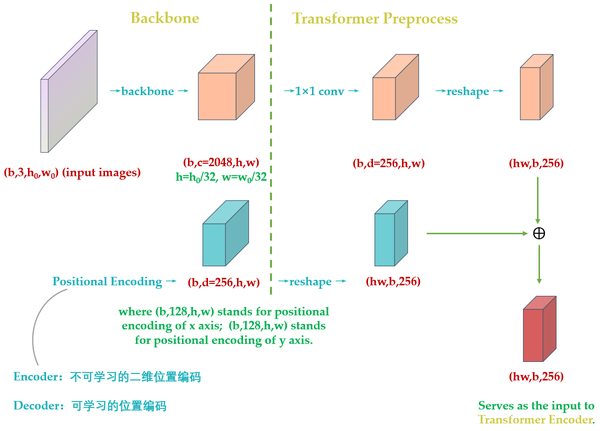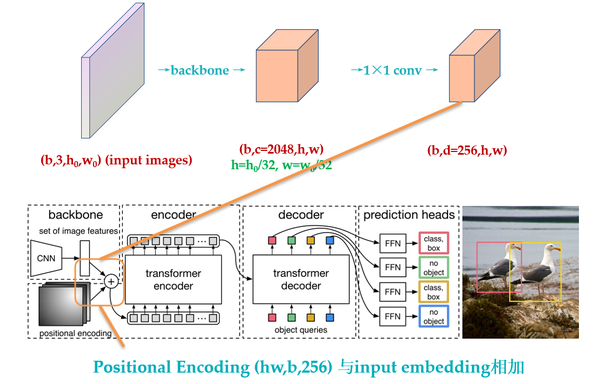Encoder最终输出的是 $(H\cdot W,b,256)$ 维的编码矩阵Embedding，按照原版Transformer的做法，把这个东西给Decoder。

• 输入编码器的位置编码需要考虑2-D空间位置。
• 位置编码向量需要加入到每个Encoder Layer中。
• 在编码器内部位置编码Positional Encoding仅仅作用于Query和Key，即只与Query和Key相加，Value不做任何处理。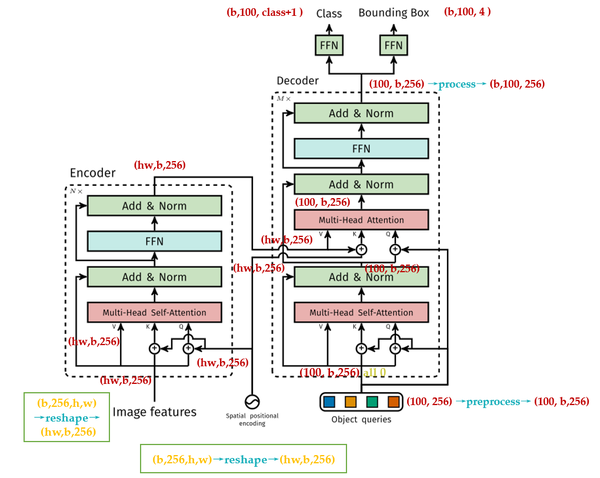3 再看decoder：

DETR的Decoder和原版Transformer的decoder是不太一样的，如下图30和19所示。

DETR的Decoder主要有两个输入：

1. Transformer Encoder输出的Embedding与 position encoding 之和。
2. Object queries。

Object queries是一个维度为 $(100,b,256)$ 维的张量，数值类型是nn.Embedding，说明这个张量是可以学习的，即：我们的Object queries是可学习的。Object queries矩阵内部通过学习建模了100个物体之间的全局关系，例如房间里面的桌子旁边(A类)一般是放椅子(B类)，而不会是放一头大象(C类)，那么在推理时候就可以利用该全局注意力更好的进行解码预测输出。

Decoder的输入一开始也初始化成维度为 $(100,b,256)$ 维的全部元素都为0的张量，和Object queries加在一起之后充当第1个multi-head self-attention的Query和Key。第一个multi-head self-attention的Value为Decoder的输入，也就是全0的张量。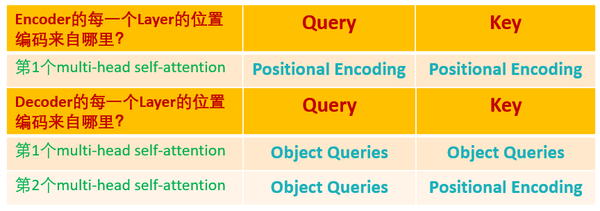$$\color{indianred}{\text{损失函数部分解读:}}$$

$$\begin{equation} \label{eq:matching} \hat{\sigma} = \arg\min_{\sigma\in\SigmaN} \sum{i}^{N} L_{match}(yi, \hat y{\sigma(i)}), \end{equation} \tag{12}$$

$$-\mathbb{1}_{\left{ ci\neq\varnothing \right}}\hat p{\sigma(i)}(ci) + \mathbb{1}{\left{ ci\neq\varnothing \right}} L{box}({b{i}, \hat b{\sigma(i)}}) \tag{13}$$

$$L{\text{Hungarian}}({y, \hat y}) = \sum{i=1}^N \left[-\log \hat p{\hat{\sigma}(i)}(c{i}) + \mathbb{1}_{\left{ ci\neq\varnothing \right}} \ L{box}{(b{i}, \hat b{\hat{\sigma}(i)}})\right] \tag{14}$$

$$L{box}{(b{i}, \hat b{\hat{\sigma}(i)}}) = \lambda{\rm iou}L{iou}({b{i}, \hat b{\sigma(i)}})+ \lambda{\rm L1}||b{i}- \hat b{\sigma(i)}||1 ,\; where \;\lambda{\rm iou}, \lambda_{\rm L1}\in R \tag{15}$$

Hungarian意思就是匈牙利，也就是前面的 $L_{match}$ ，上述意思是需要计算 $M$ 个 $\text{GT}\;\color{purple}{\text{Bounding Box}}$ 和 $N$ 个输预测出集合两两之间的广义距离，距离越近表示越可能是最优匹配关系，也就是两者最密切。广义距离的计算考虑了分类分支和回归分支。

DETR是怎么训练的？

$$(\text{label}{\color{orange}{\text{Car}}}=3,\text{label}{\color{green}{\text{Dog}}}=24,\text{label}_{\color{orange}{\color{darkturquoise}{\text{Horse}}}}=75)\$$

linear_sum_assignment

"匈牙利算法 (Hungarian Algorithm)"

Query可以视作代表不同 $\text{Object}$ 的信息，而Key和Value可以视作代表图像的全局信息。

，则这个特征就能提取出来，最后通过2个
$FFN$

Experiments：

1. 性能对比：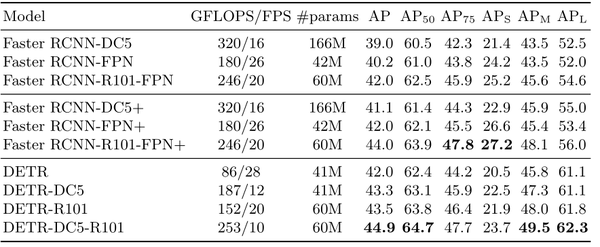2. 编码器层数对比实验：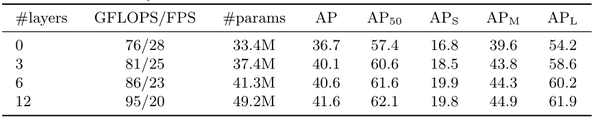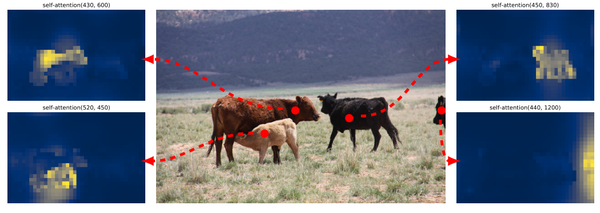3. 解码器层数对比实验：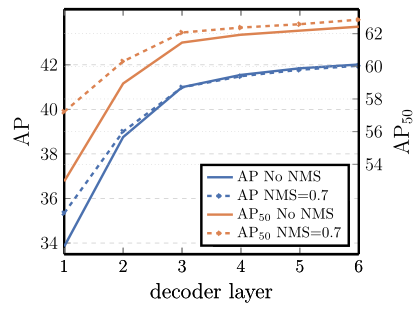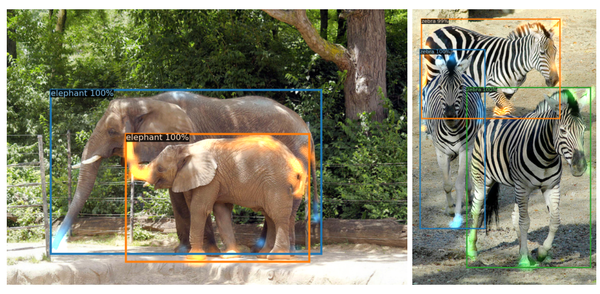• 3.2 DETR代码解读：

DETR的二维位置编码：

x：(b,c,H,W)

self.num_pos_feats = 128

pos_x：(b,H,W,128)
pos_y：(b,H,W,128)
flatten后面的数字指的是：flatten()方法应从哪个轴开始展开操作。
torch.stack((pos_x[:, :, :, 0::2].sin(), pos_x[:, :, :, 1::2].cos()), dim=4).flatten(3)
pos_y = torch.stack((pos_y[:, :, :, 0::2].sin(), pos_y[:, :, :, 1::2].cos()), dim=4)

torch.cat((pos_y, pos_x), dim=3)之后变为(b,H,W,256)，再最后permute为(b,256，H,W)。
PositionEmbeddingSine类继承nn.Module类。

class PositionEmbeddingSine(nn.Module):

def __init__(self, num_pos_feats=64, temperature=10000, normalize=False, scale=None):
super().__init__()
self.num_pos_feats = num_pos_feats
self.temperature = temperature
self.normalize = normalize
if scale is not None and normalize is False:
raise ValueError("normalize should be True if scale is passed")
if scale is None:
scale = 2 * math.pi
self.scale = scale

def forward(self, tensor_list: NestedTensor):
#输入是b,c,h,w
#用于表示动态shape，是pytorch中tensor新特性https://github.com/pytorch/nestedtensor
x = tensor_list.tensors
# 因为图像是2d的，所以位置编码也分为x,y方向
# 1 1 1 1 ..  2 2 2 2... 3 3 3...
# 1 2 3 4 ... 1 2 3 4...
if self.normalize:
eps = 1e-6
y_embed = y_embed / (y_embed[:, -1:, :] + eps) * self.scale
x_embed = x_embed / (x_embed[:, :, -1:] + eps) * self.scale

# num_pos_feats = 128
# 0~127 self.num_pos_feats=128,因为前面输入向量是256，编码是一半sin，一半cos
dim_t = torch.arange(self.num_pos_feats, dtype=torch.float32, device=x.device)
dim_t = self.temperature **
(2 * (dim_t // 2) / self.num_pos_feats)

# 输出shape=b,h,w,128
pos_x = x_embed[:, :, :, None] / dim_t
pos_y = y_embed[:, :, :, None] / dim_t
pos_x = torch.stack((pos_x[:, :, :, 0::2].sin(), pos_x[:, :, :, 1::2].cos()), dim=4).flatten(3)
pos_y = torch.stack((pos_y[:, :, :, 0::2].sin(), pos_y[:, :, :, 1::2].cos()), dim=4).flatten(3)
pos = torch.cat((pos_y, pos_x), dim=3).permute(0, 3, 1, 2)
# 每个特征图的xy位置都编码成256的向量，其中前128是y方向编码，而128是x方向编码
return pos
# b,n=256,h,w

NestedTensor：

to()函数：把变量移到GPU中。

Backbone：

class BackboneBase(nn.Module):

def __init__(self, backbone: nn.Module, train_backbone: bool, num_channels: int, return_interm_layers: bool):
super().__init__()
for name, parameter in backbone.named_parameters():
if not train_backbone or 'layer2' not in name and 'layer3' not in name and 'layer4' not in name:
if return_interm_layers:
return_layers = {"layer1": "0", "layer2": "1", "layer3": "2", "layer4": "3"}
else:
return_layers = {'layer4': "0"}

#作用的模型：定义BackboneBase时传入的nn.Moduleclass的backbone，返回的layer：来自bool变量return_interm_layers
self.body = IntermediateLayerGetter(backbone, return_layers=return_layers)
self.num_channels = num_channels

def forward(self, tensor_list: NestedTensor):
#BackboneBase的输入是一个NestedTensor
#xs中间层的输出，
xs = self.body(tensor_list.tensors)
out: Dict[str, NestedTensor] = {}
for name, x in xs.items():
assert m is not None
return out

class Backbone(BackboneBase):
"""ResNet backbone with frozen BatchNorm."""
def __init__(self, name: str,
train_backbone: bool,
return_interm_layers: bool,
dilation: bool):
#根据name选择backbone, num_channels, return_interm_layers等，传入BackboneBase初始化
backbone = getattr(torchvision.models, name)(
replace_stride_with_dilation=[False, False, dilation],
pretrained=is_main_process(), norm_layer=FrozenBatchNorm2d)
num_channels = 512 if name in ('resnet18', 'resnet34') else 2048
super().__init__(backbone, train_backbone, num_channels, return_interm_layers)

Backbone完以后输出(b,c,h,w)，再经过PositionEmbeddingSine输出(b,H,W,256)。

class Joiner(nn.Sequential):
def __init__(self, backbone, position_embedding):
super().__init__(backbone, position_embedding)

def forward(self, tensor_list: NestedTensor):
xs = self(tensor_list)
out: List[NestedTensor] = []
pos = []
for name, x in xs.items():
out.append(x)
# position encoding
pos.append(self(x).to(x.tensors.dtype))

return out, pos

def build_backbone(args):
#position_embedding是个nn.module
position_embedding = build_position_encoding(args)
train_backbone = args.lr_backbone > 0
#backbone是个nn.module
backbone = Backbone(args.backbone, train_backbone, return_interm_layers, args.dilation)
#nn.Sequential在一起
model = Joiner(backbone, position_embedding)
model.num_channels = backbone.num_channels
return model

Transformer的一个Encoder Layer：

class TransformerEncoderLayer(nn.Module):

def __init__(self, d_model, nhead, dim_feedforward=2048, dropout=0.1,
activation="relu", normalize_before=False):
super().__init__()
# Implementation of Feedforward model
self.linear1 = nn.Linear(d_model, dim_feedforward)
self.dropout = nn.Dropout(dropout)
self.linear2 = nn.Linear(dim_feedforward, d_model)

self.norm1 = nn.LayerNorm(d_model)
self.norm2 = nn.LayerNorm(d_model)
self.dropout1 = nn.Dropout(dropout)
self.dropout2 = nn.Dropout(dropout)

self.activation = _get_activation_fn(activation)
self.normalize_before = normalize_before

def with_pos_embed(self, tensor, pos: Optional[Tensor]):
return tensor if pos is None else tensor + pos

def forward_post(self,
src,
pos: Optional[Tensor] = None):
# 和标准做法有点不一样，src加上位置编码得到q和k，但是v依然还是src，
# 也就是v和qk不一样
q = k = self.with_pos_embed(src, pos)
src = src + self.dropout1(src2)
src = self.norm1(src)
#FFN
src2 = self.linear2(self.dropout(self.activation(self.linear1(src))))
src = src + self.dropout2(src2)
src = self.norm2(src)
return src

def forward_pre(self, src,
pos: Optional[Tensor] = None):
src2 = self.norm1(src)
q = k = self.with_pos_embed(src2, pos)
src = src + self.dropout1(src2)
src2 = self.norm2(src)
src2 = self.linear2(self.dropout(self.activation(self.linear1(src2))))
src = src + self.dropout2(src2)
return src

def forward(self, src,
pos: Optional[Tensor] = None):
if self.normalize_before:
return self.forward_post(src, src_mask, src_key_padding_mask, pos)

class TransformerEncoder(nn.Module):
def __init__(self, encoder_layer, num_layers, norm=None):
super().__init__()
# 编码器copy6份
self.layers = _get_clones(encoder_layer, num_layers)
self.num_layers = num_layers
self.norm = norm

def forward(self, src,
pos: Optional[Tensor] = None):
# 内部包括6个编码器，顺序运行
# src是图像特征输入，shape=hxw,b,256
output = src
for layer in self.layers:
# 每个编码器都需要加入pos位置编码
# 第一个编码器输入来自图像特征，后面的编码器输入来自前一个编码器输出
return output

Object Queries：可学习的位置编码：

class PositionEmbeddingLearned(nn.Module):
"""
Absolute pos embedding, learned.
"""
def __init__(self, num_pos_feats=256):
super().__init__()]
#这里使用了nn.Embedding，这是一个矩阵类，里面初始化了一个随机矩阵，矩阵的长是字典的大小，宽是用来表示字典中每个元素的属性向量，
# 向量的维度根据你想要表示的元素的复杂度而定。类实例化之后可以根据字典中元素的下标来查找元素对应的向量。输入下标0，输出就是embeds矩阵中第0行。
self.row_embed = nn.Embedding(50, num_pos_feats)
self.col_embed = nn.Embedding(50, num_pos_feats)
self.reset_parameters()

def reset_parameters(self):
nn.init.uniform_(self.row_embed.weight)
nn.init.uniform_(self.col_embed.weight)

#输入依旧是NestedTensor
def forward(self, tensor_list: NestedTensor):
x = tensor_list.tensors
h, w = x.shape[-2:]
i = torch.arange(w, device=x.device)
j = torch.arange(h, device=x.device)

#x_emb：(w, 128)
#y_emb：(h, 128)
x_emb = self.col_embed(i)
y_emb = self.row_embed(j)
pos = torch.cat([
x_emb.unsqueeze(0).repeat(h, 1, 1),#(1,w,128) → (h,w,128)
y_emb.unsqueeze(1).repeat(1, w, 1),#(h,1,128) → (h,w,128)
], dim=-1).permute(2, 0, 1).unsqueeze(0).repeat(x.shape, 1, 1, 1)
#(h,w,256) → (256,h,w) → (1,256,h,w) → (b,256,h,w)
return pos

def build_position_encoding(args):
N_steps = args.hidden_dim // 2
if args.position_embedding in ('v2', 'sine'):
# TODO find a better way of exposing other arguments
position_embedding = PositionEmbeddingSine(N_steps, normalize=True)
elif args.position_embedding in ('v3', 'learned'):
position_embedding = PositionEmbeddingLearned(N_steps)
else:
raise ValueError(f"not supported {args.position_embedding}")

return position_embedding

Transformer的一个Decoder Layer：

object queries(query_pos)
Encoder的位置编码(pos)
Encoder的输出(memory)

    def forward_post(self, tgt, memory,
pos: Optional[Tensor] = None,
query_pos: Optional[Tensor] = None):

#query,key的输入是object queries(query_pos) + Decoder的输入(tgt),shape都是(100,b,256)
#value的输入是Decoder的输入(tgt),shape = (100,b,256)
q = k = self.with_pos_embed(tgt, query_pos)

tgt = tgt + self.dropout1(tgt2)
tgt = self.norm1(tgt)

#query的输入是上一个attention的输出(tgt) + object queries(query_pos)
#key的输入是Encoder的位置编码(pos) + Encoder的输出(memory)
#value的输入是Encoder的输出(memory)
key=self.with_pos_embed(memory, pos),

tgt = tgt + self.dropout2(tgt2)
tgt = self.norm2(tgt)

#FFN
tgt2 = self.linear2(self.dropout(self.activation(self.linear1(tgt))))
tgt = tgt + self.dropout3(tgt2)
tgt = self.norm3(tgt)
return tgt

def forward_pre(self, tgt, memory,
pos: Optional[Tensor] = None,
query_pos: Optional[Tensor] = None):
tgt2 = self.norm1(tgt)
q = k = self.with_pos_embed(tgt2, query_pos)
tgt = tgt + self.dropout1(tgt2)
tgt2 = self.norm2(tgt)
key=self.with_pos_embed(memory, pos),
tgt = tgt + self.dropout2(tgt2)
tgt2 = self.norm3(tgt)
tgt2 = self.linear2(self.dropout(self.activation(self.linear1(tgt2))))
tgt = tgt + self.dropout3(tgt2)
return tgt

def forward(self, tgt, memory,
pos: Optional[Tensor] = None,
query_pos: Optional[Tensor] = None):
if self.normalize_before:
tgt_key_padding_mask, memory_key_padding_mask, pos, query_pos)

class TransformerDecoder(nn.Module):

#值得注意的是：在使用TransformerDecoder时需要传入的参数有：
def forward(self, tgt, memory,
pos: Optional[Tensor] = None,
query_pos: Optional[Tensor] = None):
output = tgt

intermediate = []

for layer in self.layers:
pos=pos, query_pos=query_pos)
if self.return_intermediate:
intermediate.append(self.norm(output))

if self.norm is not None:
output = self.norm(output)
if self.return_intermediate:
intermediate.pop()
intermediate.append(output)

if self.return_intermediate:

return output.unsqueeze(0)

class MLP(nn.Module):
""" Very simple multi-layer perceptron (also called FFN)"""

predictions比targets的数量多，但我们要进行1-to-1 matching，所以多的predictions将与 $\varnothing$ 匹配。

m包含了这个batch内部所有的 $\text{GT}\;\color{purple}{\text{Bounding Box}}$ 。

# pred_logits:[b,100,92]
# pred_boxes:[b,100,4]
# targets是个长度为b的list，其中的每个元素是个字典，共包含：labels-长度为(m,)的Tensor，元素是标签；boxes-长度为(m,4)的Tensor，元素是Bounding Box。
# detr分类输出，num_queries=100，shape是(b,100,92)
bs, num_queries = outputs["pred_logits"].shape[:2]

# We flatten to compute the cost matrices in a batch
out_prob = outputs["pred_logits"].flatten(0, 1).softmax(-1)  # [batch_size * num_queries, num_classes] = [100b, 92]
out_bbox = outputs["pred_boxes"].flatten(0, 1)  # [batch_size * num_queries, 4] = [100b, 4]

# 准备分类target shape=(m,)里面存储的是类别索引，m包括了整个batch内部的所有gt bbox
# Also concat the target labels and boxes
tgt_ids = torch.cat([v["labels"] for v in targets])# (m,)[3,6,7,9,5,9,3]
# 准备bbox target shape=(m,4)，已经归一化了
tgt_bbox = torch.cat([v["boxes"] for v in targets])# (m,4)

#(100b,92)->(100b, m)，对于每个预测结果，把目前gt里面有的所有类别值提取出来，其余值不需要参与匹配
#对应上述公式，类似于nll loss，但是更加简单
# Compute the classification cost. Contrary to the loss, we don't use the NLL,
# but approximate it in 1 - proba[target class].
# The 1 is a constant that doesn't change the matching, it can be ommitted.
#行：取每一行；列：只取tgt_ids对应的m列
cost_class = -out_prob[:, tgt_ids]# (100b, m)

# Compute the L1 cost between boxes, 计算out_bbox和tgt_bbox两两之间的l1距离 (100b, m)
cost_bbox = torch.cdist(out_bbox, tgt_bbox, p=1)# (100b, m)

# Compute the giou cost betwen boxes, 额外多计算一个giou loss (100b, m)
cost_giou = -generalized_box_iou(box_cxcywh_to_xyxy(out_bbox), box_cxcywh_to_xyxy(tgt_bbox))

#得到最终的广义距离(100b, m)，距离越小越可能是最优匹配
# Final cost matrix
C = self.cost_bbox * cost_bbox + self.cost_class * cost_class + self.cost_giou * cost_giou
#(100b, m)--> (b, 100, m)
C = C.view(bs, num_queries, -1).cpu()

#计算每个batch内部有多少物体，后续计算时候按照单张图片进行匹配，没必要batch级别匹配,徒增计算
sizes = [len(v["boxes"]) for v in targets]
#匈牙利最优匹配，返回匹配索引
#enumerate(C.split(sizes, -1))]：(b,100,image1,image2,image3,...)
indices = [linear_sum_assignment(c[i]) for i, c in enumerate(C.split(sizes, -1))]
return [(torch.as_tensor(i, dtype=torch.int64), torch.as_tensor(j, dtype=torch.int64)) for i, j in indices]